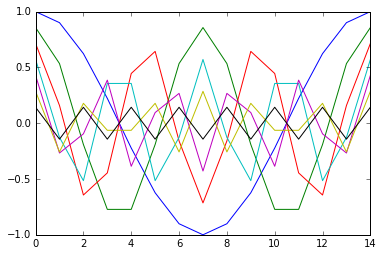# Reading and Writing Audio Files with audioread¶

back to overview page

The module audioread can be found there: https://github.com/sampsyo/audioread

This isn't really an option because it has the same disadvantages as the wave module (and more) and it has to be installed manually (but at least it's available as Debian package).

• WAVEX seems to work
• seems to work for both Python 2 and 3

• always 16-bit values are returned (even if file has different data, e.g. pcm32, float32)
• manual de-interleaving and conversion is necessary
• the number of channels is wrong in some cases (it should work for 1 and 2-channel files though)
• no error/warning messages if something goes wrong

I don't recommend the module audioread, but you can have a look how it is supposed to work, anyway.

First, let's enable inline plotting and load the NumPy stuff:

In :
%matplotlib inline
import matplotlib.pyplot as plt
import numpy as np


Following code should read a 16-bit WAV file.

To convert it to floating point values and to normalize to a range from -1 to 1, we're using the function pcm2float(), located in the file utility.py.

In :
import audioread
import utility

temp = bytearray()

with audioread.audio_open('data/test_wav_pcm16.wav') as f:
framerate = f.samplerate
duration = f.duration
nchannels = f.channels
print("sampling rate = {framerate} Hz, duration = {duration} seconds, channels = {nchannels}".format(**locals()))

# chunk size can be specified with 'block_samples' (default 1024):
for chunk in f.read_data():
temp.extend(chunk)

sig = np.frombuffer(temp, dtype='<i2').reshape(-1, nchannels)

normalized = utility.pcm2float(sig, np.float32)
plt.plot(normalized);

sampling rate = 44100 Hz, duration = 0.0003401360544217687 seconds, channels = 7TODO

## Version Info¶

In :
import sys, numpy, IPython
print("Versions: NumPy = {}; IPython = {}".format(numpy.__version__, IPython.__version__))

print("Python interpreter:")
print(sys.version)

try:
except:
import traceback
traceback.print_exc()

Versions: NumPy = 1.9.2; IPython = 3.2.0
Python interpreter:
3.4.3+ (default, Oct 10 2015, 09:15:38)
[GCC 5.2.1 20151003]

Traceback (most recent call last):
File "<ipython-input-3-3fe2051c64a7>", line 8, in <module>

<p xmlns:dct="http://purl.org/dc/terms/">